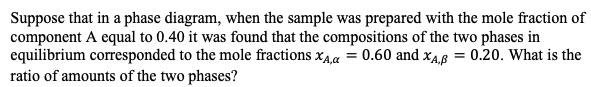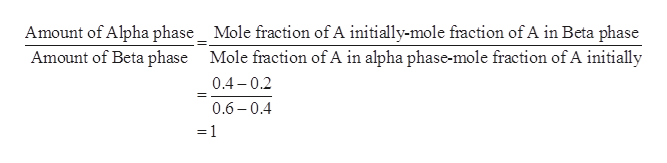# Suppose that in a phase diagram, when the sample was prepared with the mole fraction ofcomponent A equal to 0.40 it was found that the compositions of the two phases inequilibrium corresponded to the mole fractions xAa 0.60 and xA 0.20. What is theratio of amounts of the two phases?

Questionhelp_outlineImage TranscriptioncloseSuppose that in a phase diagram, when the sample was prepared with the mole fraction of component A equal to 0.40 it was found that the compositions of the two phases in equilibrium corresponded to the mole fractions xAa 0.60 and xA 0.20. What is the ratio of amounts of the two phases? fullscreen
check_circle

Step 1

The lever rule can be defined as a rule which is used for the determination of the mole fraction of each phase of a binary equilibrium phase diagram. It can also be used to estimate the fraction of the liquid and solid phases for a given binary composition and the temperature which is present between liquidus and solidus line.

Step 2

The mole fractions are given to be as follows:

Step 3

The mole fraction of A initially is given to be 0.40.

Using the lever rule, the ...help_outlineImage TranscriptioncloseAmount of Alpha phase_ Mole fraction of A initially-mole fraction of A in Beta phase Amount of Beta phase Mole fraction of A in alpha phase-mole fraction of A initially 0.4 0.2 0.6 0.4 =1 fullscreen

### Want to see the full answer?

See Solution

#### Want to see this answer and more?

Solutions are written by subject experts who are available 24/7. Questions are typically answered within 1 hour.*

See Solution
*Response times may vary by subject and question.
Tagged in

### Physical Chemistry